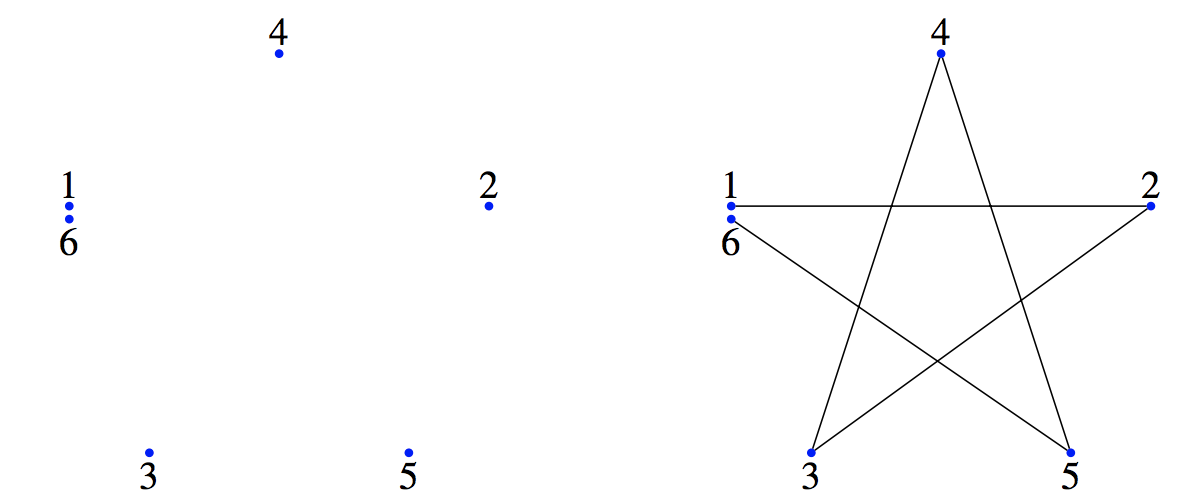시간 제한메모리 제한제출정답맞힌 사람정답 비율
2 초 512 MB51125.000%

## 문제

Have you ever played around with connect-the-dots? For those of us who are not artistic, this is one of the only ways to draw amazing pictures. For a connect-the-dots, there are n dots on the page, each labelled with an integer between 1 and n. You are to draw n − 1 straight lines: one between 1 and 2, one between 2 and 3, one between 3 and 4, and so on. In the end, the image you have should be beautiful. Here is an example before and after you connect the dots:Today, you are going to (hopefully) help me draw a connect-the-dots on the surface of a balloon. For simplicity, we are going to assume that the balloon is a perfect sphere. To begin with, the balloon has a radius of 1 centimetre. I have drawn n dots on the surface of the balloon and labelled each of them from 1 to n. As I inflate the balloon, the points will start moving further and further apart. My goal is to end up with as large a picture as possible (that is, the total length of the n − 1 line segments should be as large as possible).

Because I do not want the image to look faded, I will first inflate the balloon and then (and only then) draw the n−1 lines. I can increase the volume of the balloon by v cm3 per minute and I can draw at a speed of d cm per minute. When I draw the line from point i to i + 1, I will draw a straight line along the surface of the balloon. Each line will be the shortest line between the two points (in certain cases, there may be multiple shortest lines—which one is drawn is not important for this problem since we are only concerned with the lengths of the lines).

I have T total minutes to spare, so the time to both inflate the balloon and draw the lines must be at most T minutes. When I finish drawing, all of the n − 1 lines must be fully drawn (completed). What is the largest sum of line lengths that I can draw?

## 입력

The input starts with a line containing four integers n, T, v and d, where n (2 ≤ n ≤ 1 000) is the number of points, T (1 ≤ T ≤ 1 000 000) is the time available to both inflate the balloon and draw the n − 1 lines, v (1 ≤ v ≤ 1 000) is the speed in which I can inflate the balloon (in cm3 per minute) and d (1 ≤ d ≤ 1 000) is the speed in which I can draw a line (in cm per minute).

The next n lines describe the points on the balloon in order from 1 to n, assuming that the balloon’s centre is at the origin. Each of these lines consists of two real numbers x and y and an integer s (s ∈ {−1, 1}), giving the point’s position on the surface of the ballon at (x, y, z), where z = s√(1 − x2 − y2). It is guaranteed that 0 ≤ x2 + y2 ≤ 1 and x and y are in centimetres.

Note that lines may intersect one another (or may overlap completely), and so you may count a certain line segment multiple times (see Sample Input 1 for example). Each x and y will be given to no more than 6 digits of precision. It is guaranteed that I can draw the image on the balloon of radius one in at most T minutes.

## 출력

Display the length (in centimetres) of the largest completed drawing on the balloon that I can make. Your answer should have an absolute or relative error of less than 10−6.

## 예제 입력 1

3 10 10 20
1 0 1
0 1 1
1 0 1


## 예제 출력 1

9.03600166336021


## 예제 입력 2

2 10 1 1
0.3 0.5 1
0.2 0.4 -1


## 예제 출력 2

2.86454577269227


## 예제 입력 3

3 10 1 1
0.3 0.5 1
0.3 0.5 1
0.2 0.4 -1


## 예제 출력 3

2.86454577269227


## 힌트

Be careful when x2 + y2 = 1. When trying to evaluate √(1 − x2 − y2), the value of 1 − x2 − y2 may be stored as −0.000 · · · 0001, so √(1 − x2 − y2) may fail (you cannot take the square root of a negative number).